# Understand the difference between int and Integer and BigInteger in java and float and double

The other day, when I asked the past question of the programming contest with haskell, when I wrote the logic to manage the exponentiation, I wanted the final result to be `12340000`, but it didn't pass because it was` 12340000.0`. It's embarrassing.

It's embarrassing, but listening is also a commentary on shame for a lifetime, and what you don't know can be done if you learn and master it obediently. Can't you?

I'm not sure, but in short, it's a story about putting together things like ʻint` that I used in an ambiguous way.

## Light background

I usually make a contract management system with java / DDD, but the numbers I handle are only tens of thousands of integers. It's a little yen, counting the number of contracts, and so on. There is no daily billing for the month. I'm surprised.

Thanks to DDD's value object, I don't often touch raw ʻint`s.

So, just before writing this article, for example, the level of understanding of java is like this.

"` Byte`? I don't know, but I'm scared. " "` Long`? "` BigInteger`? Big So ~ " "` Float`? Fluffy ~ " "` Double`? What is double ~?

The degree of understanding. This is serious.

(I only knew that double Italian was doppio)

So just acknowledge that that many people put it together!

## Thank you for the haskell version

Originally I checked it with haskell, but I thought that it would be better to check some languages, so I also tried it with java.

I checked it a little with python, but I think I'll use haskell in my book and java used at work.

So, thank you for your cooperation. → Understand the difference between Haskell Int and Integer, Float, Double and Rational

## First overview

Before we get into programming, let's talk about math.

Even if you say mathematics, scary things like algebra and category theory do not come out. I'm scared too. It's about junior high school.

Look at this first.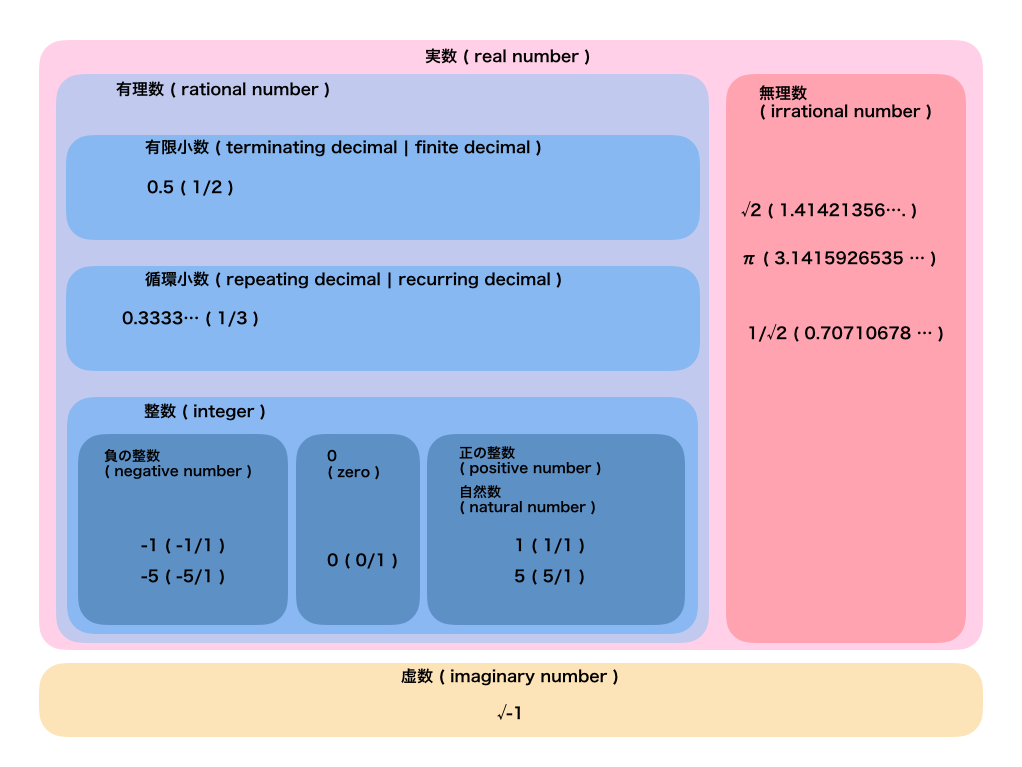I will explain it roughly.

### Real and imaginary

The numbers you usually see are usually `real numbers`.

On the other hand, `imaginary number` is a number invented because it is convenient, but it does not exist in reality. A typical example is `√-1` or it is expressed as ʻi`. It's easy to understand. I'm not sure.

The `imaginary number` is ** a number that squares to a real number less than 0 **, and the` real number` is defined as ** otherwise **.

I don't want to think about it in detail, so it's okay to use "about a real number".

### Rational and irrational numbers

The classification of `real numbers` is taken seriously.

`Real numbers` are roughly divided into`rational numbers`and` irrational numbers`, but `rational numbers` are ** numbers that can be expressed by the ratio of integers **.

On the other hand, other numbers ** that cannot be expressed by the ratio of integers are called `irrational numbers`.

### Integers, finite decimals and recurring decimals

Both are `rational numbers`.

There is no need to explain `integer`. `3` can be expressed as` 3/1`, so it is a `rational number`.

A finite decimal is a `decimal with an end, such as` 0.5`. It can be expressed as a ratio of `integer` such as` 1/2`.

On the other hand, `decimal numbers` such as`0.333 ...`and`0.142857142857142857 ...`, in which the same number is repeated indefinitely, are called` recurring decimals`. This can also be expressed as a ratio of `integers` such as` 1/3` and `1/7`.

### Negative integers, positive integers, zeros and natural numbers

`Integer` is the most familiar, so I don't think it's a problem, but for the time being.

The `negative integer` is` -1` or `-5` and can be expressed in the form of` -5/1`.

`0` is` 0/1`, isn't it?

The same is true for `positive integers`.

Also, a `positive integer` is called a` natural number`. (It doesn't matter if you include `0` in this article)

### Decimal and fraction

A supplement for `decimal`s and` fractions`.

`Fraction` is a number expressed by ** ratio of numbers **, and at first glance it seems to be the same as` rational number`. However, since `rational numbers` are ** ratios of integers **, fractions are a broader concept.

For example, there is `1 / √2`. This is a `irrational number` because it is not a ** integer ratio **. (Since it is about 0.7, it is about `0.5` when squared, which is larger than` 0`, so it is not a `imaginary number`.

Also, for example, there is a `infinite decimal`, but in the previous figure, both the`recurring decimal`and the` irrational decimal` of the `rational number` are` infinite decimals`. It's the difference between circulating and non-circulating.

## English you want to keep

While keeping the above points in mind, we programmers need to know English words, so we will summarize them roughly. (Although English is also included in the picture.)

`Real number` is` real number` and `imaginary number` is ʻimaginary number`, so it's easy to get an image.

I'm not very familiar with it, but the `rational number` is the` rational number`. If you write it in copy, you will rarely hit it.

Since `ratio` means` ratio`, some people may have used it in variable names.

Also, although not in the picture, `decimal` is` decimal` and `fraction` is` fraction`.

## To the world of programming (java)

I'd like to see the sample code in java at once, but there are big differences between the human world and the computer world.

That is, "memory is finite".

Where it is related is, for example, "great deke number" and "infinite decimal number".

### Fixed length integer

For example, java's ʻint`is a 32-bit fixed`integer`.

Due to the limited memory of the computer, the numbers are limited to the range of 32 `0 | 1`.

### Arbitrary precision integer

On the other hand, `multi-precision integer` ** is a numerical expression method that dynamically allocates memory according to the number to be handled.

In theory, you can handle an infinite number. (Of course, as long as computer memory allows.)

### Fixed-precision integers and multiple-precision integers

This `fixed-length integer` causes the overflow that everyone loves.

For example, the `byte` in java is an 8-bit fixed` integer`. The first bit is used as the sign for positive and negative signs, and the rest is used for expressing the value.

`0000|0000`From`1`Starting to increase little by little`0111|1111`From`1000|0000`Overflow where `1111|1111`From`1|0000|0000`The 9th bit is out of range at the point where`0000|0000`Will be treated as. (`|` Is inserted every 4 digits for easy viewing.)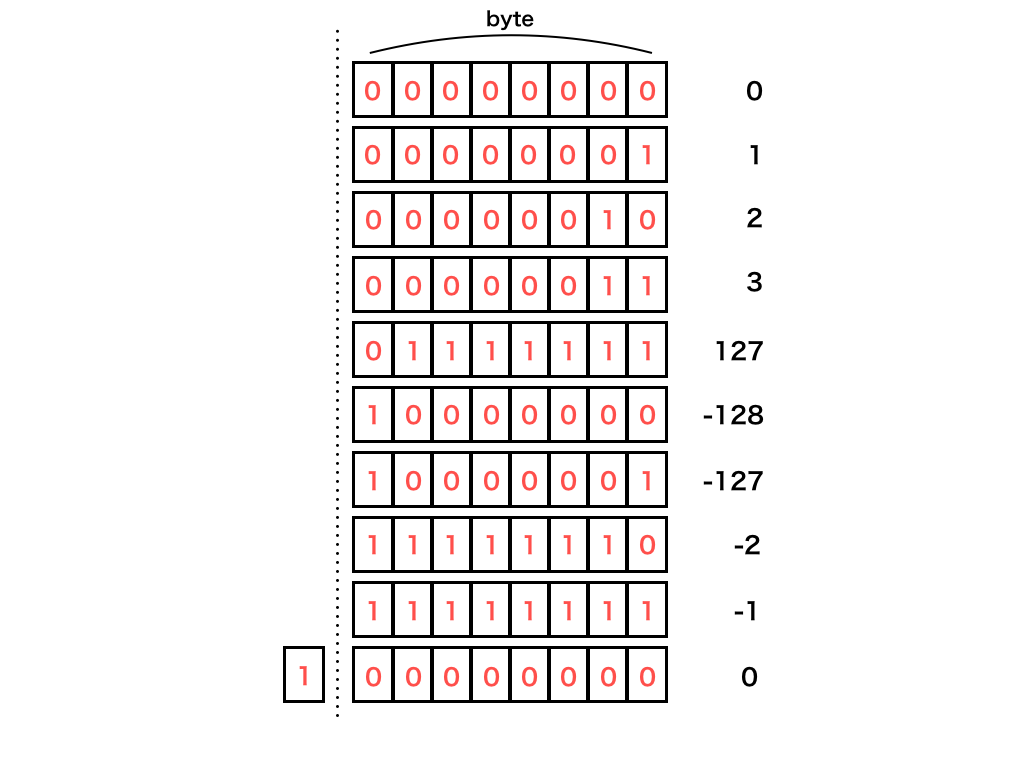On the other hand, `BigInteger` is` a multiple-precision integer`. When an overflow is about to occur, it dynamically allocates memory so it won't overflow.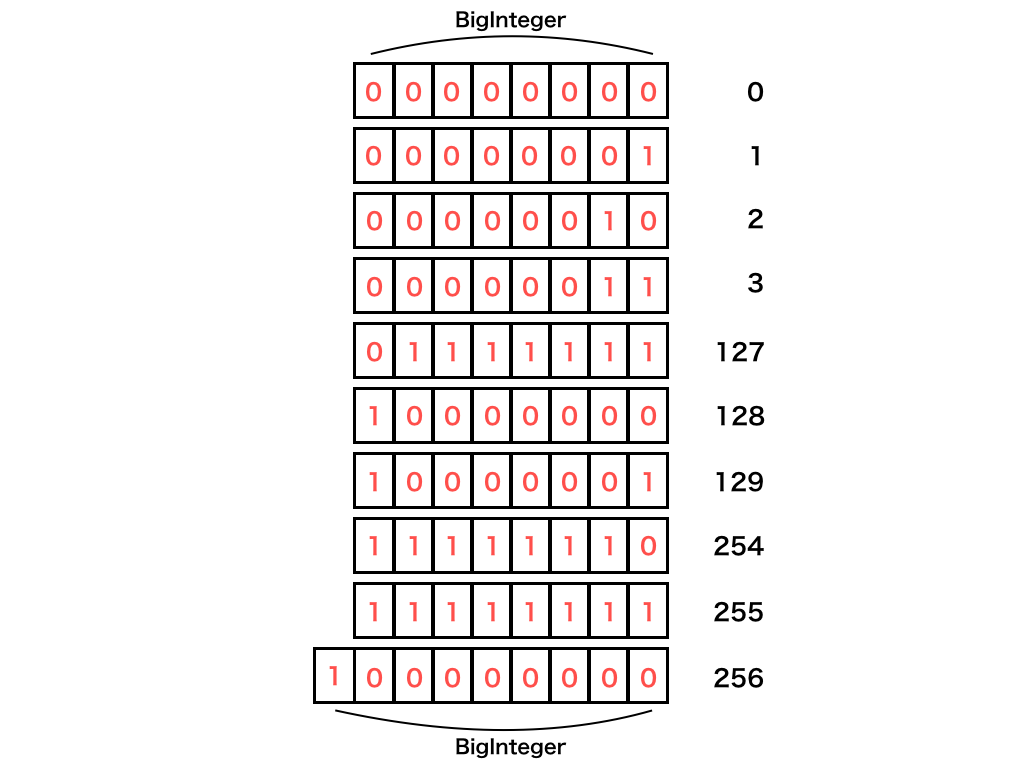(The above figure is an image because it depends on the mounting method for holding the code and value.)

`Fixed-length integers` have excellent memory efficiency and performance, and` multiple-precision integers` have excellent accuracy. These are the right people in the right place.

### Floating point

There is a similar idea for `decimal`s as well as for` integers`.

`Floating point` is one of the ** representation methods of numbers **, and is a representation method that has a` mantissa part` and a `exponent part` of` fixed length`.

Roughly speaking, it is okay to think that the `mantissa part` represents a value and the` exponent part` represents a digit.

For example, the binary number `0.00000101` is expressed as` 101 * 2 ^ -8`.

However, this can also be expressed as `10.1 * 2 ^ -7`, so it is decided that the` mantissa part` should be `1.x` in the standard called ʻIEEE754`. So it is `1.01 * 2 ^ -6`. I also write `1.01e-6`.

This is the one with ʻe` that sometimes appears when writing code. I was scared but I overcame it.

I wonder if it is called `floating point` because the position to put the decimal point changes depending on the` mantissa part` and the `exponent part`. On the other hand, the paired word is `fixed point`, which includes, for example,`integer`.

## Check with java

The introduction has become longer. From here, we will check with Gashigashi java.

type description
byte, Byte 8bit Fixed-length integer
short, Short 16bit fixed-length integer
int, Integer 32bit Fixed-length integer
long, Long 64bit fixed length integer
float, Float Single Precision Floating Point (32bit)
double, Double Double precision floating point (64bit)
BigInteger Arbitrary precision integer
BigDecimal Multiple Length Decimal

The code below omits the `System.out.println` equivalent and the comment on that line is the result.

byte, short, int, long There are many, but don't be afraid.

These are all `fixed-length integers`, and the only difference is the precision that can be expressed.

``````Byte.MAX_VALUE;       // 127
Short.MAX_VALUE;      // 32767
Integer.MAX_VALUE;    // 2147483647
Long.MAX_VALUE;       // 9223372036854775807
``````

For example, if you enter `+ 1` to the upper limit of ʻInteger`, it will overflow.

``````Integer.MAX_VALUE + 1;    // -2147483648
``````

#### Mutual conversion

And of course, casting from less accurate to more accurate is fine, but not the other way around.

``````short s = 20000;

(int) s;    // 20000
``````
``````int i = 40000;

(short) i;    // -25536
``````

### By the way, the difference between int and Integer

It's different from the original purpose, but it's unexpectedly interesting, so I'd like to say it.

In java, ʻint` is a primitive type and ʻInteger` is a class type.

The main differences are, roughly speaking, "ʻint` does not allow` null` "and" ʻint` cannot be` T` such as `List <T>`". There is no difference between ʻint` and ʻInteger` in terms of accuracy. This is important.

Also, java has a mechanism that the compiler does a good job of converting each other, so in most cases you don't have to worry too much about either.

#### Talking about memory before mutual conversion

You may not think too much about it, but I will explain the stack area and heap area very roughly.

For example, if you write code like this. (To make it easier to distinguish between ʻint` and ʻInteger` variables, ** this article uses uppercase letters at the beginning of the variable name.)

``````Integer Ia = new Integer(1);
``````

In this case, the memory looks like this.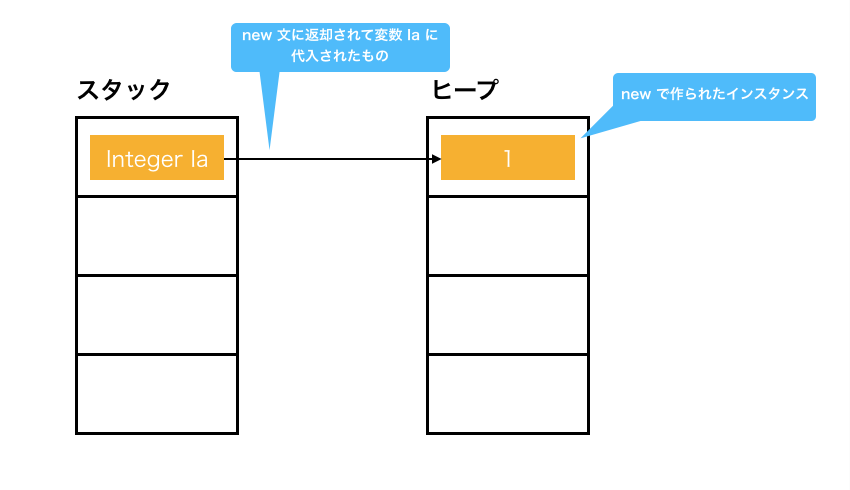If you do `new`, something will be put in the variable ʻIa` in the stack area. Somehow I feel that ʻIa` contains the instance itself, but only the ** arrow ** contains it. To put it horribly, it's a ** pointer **.

The created instance is in the heap area.

On the other hand, the primitive type ʻint` is reserved as it is in the stack area.

``````Integer Ia = new Integer(1);
Integer Ib = new Integer(1);
int ia = 1;
int ib = 1;
``````

So if you write this code, the picture will look like the one below.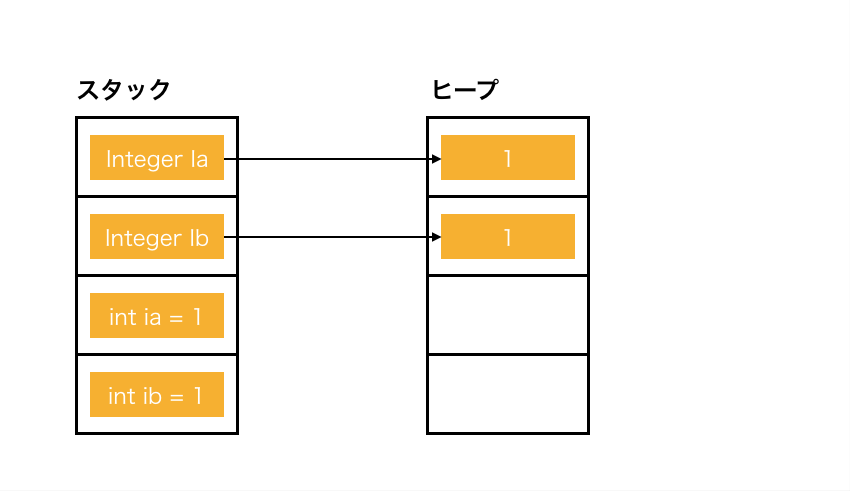#### Identity and equivalence

I'm sure there are a lot of people who have been angry with scary people saying, "Don't use`==`for comparison in java", but let's see why.

In a class type, `identity` is ** the same instance **, and` equivalence` is ** the same value **. The former is done by `==` and the latter by ʻequals`. Equivalence also depends on the implementation. (For example, when comparing DDD entities, only identity matches may be considered equivalent.)

The primitive type `==` simply compares values.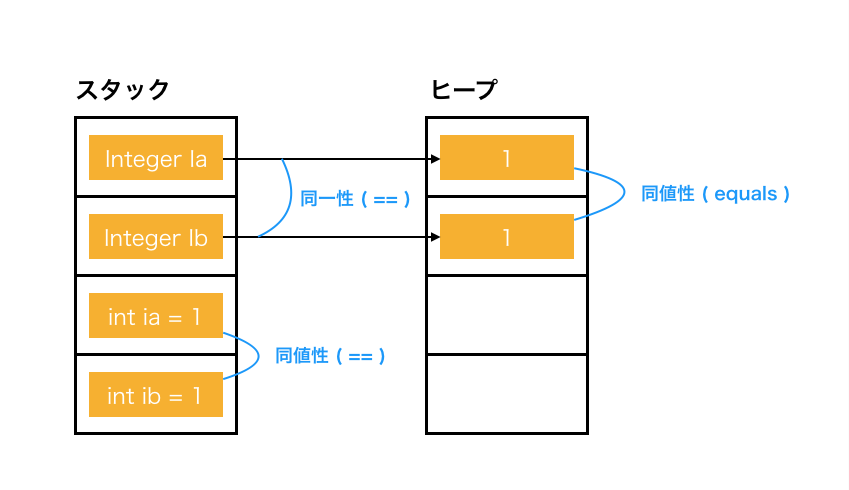So ʻIa == Ib` is ** false ** because it is an arrow with a different destination. ʻIa.equals (Ib)` is ** true ** because the destination values are the same.

For example, "Mr. A and Mr. B both have 500-yen coins, and they are ** physically different coins ** but ** have the same value **."

#### auto boxing and auto unboxing

Now that you understand the stack area, heap area, and comparison, it's about mutual conversion.

ʻInt`-> ʻInteger` is called ** boxing ** and the opposite is called ** unboxing **. I think it's an image to put in a wrapper class box.

The following code can be executed by ** auto boxing | auto unboxing **.

``````Integer Ia = new Integer(1);
int ia = Ia;                   // unboxing
``````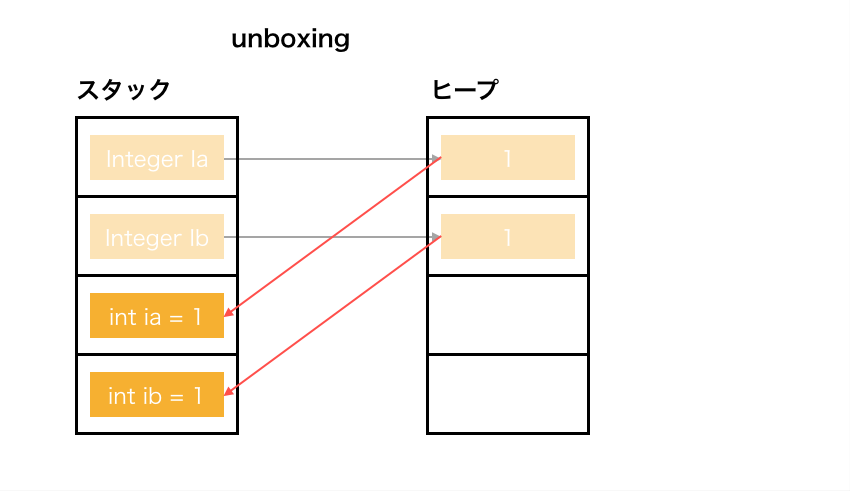``````int ib = 1;
Integer Ib = ib;               // boxing
``````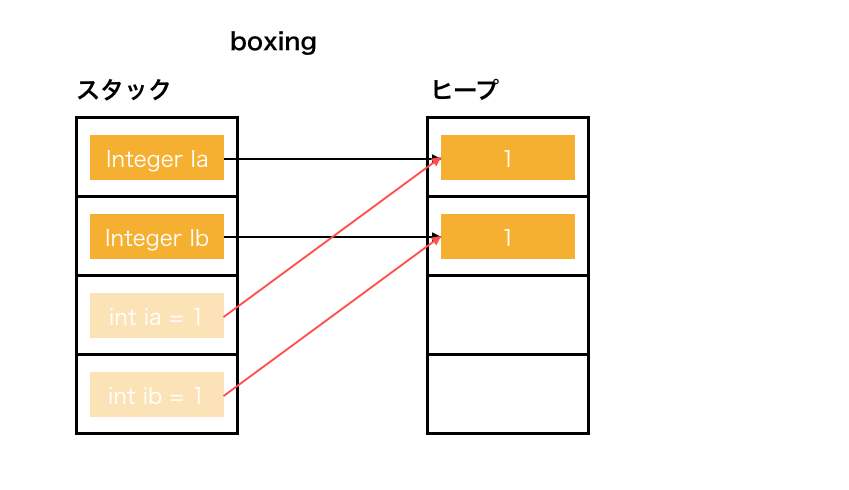Internally, values are brought to the stack area, and instances are created in the heap area to obtain references. (Actually, the original value does not disappear, but it is thin because it is easy to imagine.)

#### Digression pitfalls

Now, which of the following code would be `true` or` false`?

``````int ia = 1;
int ib = 1;
Integer Ia = ia;
Integer Ib = ib;

Ia == Ib;    // true or false ?
``````

The arrows for ʻIa` and ʻIb` should be different as they are` new` by ** auto boxing **. This is also the case in the picture above.

But this is `true`.

Apparently ** auto boxing ** is realized by ʻInteger # valueOf`, and ** auto unboxing ** is realized by ʻInteger # intValue`.

``````Integer Ia = Integer.valueOf(ia);
Integer Ib = Integer.valueOf(ib);
``````

So, the essential ʻInteger # valueOf`, but it is implemented like this.

``````public static Integer valueOf(int i) {
if (i >= IntegerCache.low && i <= IntegerCache.high)
return IntegerCache.cache[i + (-IntegerCache.low)];
return new Integer(i);
}
``````

Apparently, the frequently used `-128` ~` 127` seems to be cached. So in the above code example, it is not `new`.

With such a code, it will be `false` properly, it seems that the understanding was correct and it is safe.

``````int ia = 1000;
int ib = 1000;
Integer Ia = ia;
Integer Ib = ib;

Ia == Ib;    // false
``````

Oh, by the way, using ʻInteger # intValue` for ** auto unboxing ** internally means that if ʻIa` is` null`, ** auto unboxing ** will result in a `NullPointerException`.

#### Summary of differences between int and Integer

• Same accuracy
• Be careful when comparing
• Mutual conversion is convenient, but it doesn't completely replace it, so be careful.

That's right.

In this article, ʻint` and ʻInteger` and` float` and `Float` have no difference in accuracy, so we will use the one that is convenient for you in the sample code without notice.

float, double You've held down the `integer`. Next is the `decimal number`.

I was wondering what the `double` is, but it's clear if you study. It was said that `float` uses 32bit and` double` uses 64bit to represent the value. So double precision.

Since the memory of a computer is finite, it is impossible to completely represent a `infinite decimal number`, so it must be treated on the assumption that an error will occur.

For example, the decimal number `0.01` cannot be expressed as a finite number if it is a binary number. Since it cannot be expressed as a finite number, you have to give up somewhere, and if you repeat it, you can understand that the error will increase.

So what kind of error will occur? Let's try it.

``````float f = 0;
for (int i = 0; i < 100; i++) {
f += 0.01f;
}

double d = 0;
for (int i = 0; i < 100; i++) {
d += 0.01d;
}

f;    // 0.99999934
d;    // 1.0000000000000007
``````

`double` is closer to` 1.0`.

#### Mutual conversion

The conversion between `float` and` double`, like `short` and ʻint`, breaks when converted from the higher precision to the lower precision.

``````f;             // 0.99999934
d;             // 1.0000000000000007

(double) f;    // 0.9999993443489075
(float) d;     // 1.0
``````

If you change from `double` to` float`, it's missing.

Also, since it is finite in the first place, an error will simply occur with the following values.

``````10d / 3d;       // 3.3333333333333335
1.00000001f;    // 1.0
``````

BigInteger, BigDecimal Thank you for waiting, the `multi-length` guys.

They allocate memory dynamically according to the digits, so there is no overflow and no error. Somehow amazing.

Let's try it right away.

BigDecimal Try from the `Big Decimal` of the` decimal`. Let's deal generously with huge `integers` from the beginning.

``````BigDecimal bd = new BigDecimal(Long.MAX_VALUE);

bd;                           // 9223372036854775807

``````

Even if it is added to the upper limit of `long`, it does not overflow. It's okay to add more boldly.

``````bd.add(bd);                   // 18446744073709551614
``````

You can also add `decimal numbers`.

``````bd.add(new BigDecimal(0.5));  // 9223372036854775807.5
``````

favorite? What about the `decimal` error of?

``````BigDecimal bd = BigDecimal.ZERO;
BigDecimal x = new BigDecimal(0.01);
for (int i = 0; i < 100; i++) {
}

bd;    // 1.00000000000000002081668171172168513294309377670288085937500
``````

It's more accurate than `1.0000000000000007` of` double`. (`ToString` is made because I'm doing my best.)

What about `10d / 3d`, which has an error in` double`?

``````BigDecimal bd10 = new BigDecimal(10);
BigDecimal bd3  = new BigDecimal(3);

bd10.divide(bd3);    // ArithmeticException: Non-terminating decimal expansion; no exact representable decimal result.
``````

I saw the word `terminating` in something like Ben's figure at the beginning, and I'm angry that it's not a finite decimal.

It seems that the value with the error will not be kept with the error. It seems to be useless if you do not specify whether to cut or round up.

``````bd10.divide(bd3, RoundingMode.FLOOR) // 3

bd10.divide(bd3, RoundingMode.CEILING) // 4
``````

BigInteger This guy is easy. It is a `Big Decimal` that cannot handle` decimals`.

``````BigInteger bi = BigInteger.valueOf(Long.MAX_VALUE);

bi;            // 9223372036854775807

``````

`BigInteger` doesn't have a generation method that allows you to pass a` decimal` like` 0.5`, so it's "only this" compared to `BigDecimal`.

It's all right now. Not scary.

#### Digression: Destructive / non-destructive

By the way, if it's a java feeling, don't you feel like destroying it with ʻadd`? It's like `List # add`.

But if you understand that you may reallocate memory each time you add`, it's easy to think of creating a non-destructive, different instance each time. (It depends on the implementation method, so it may be immutable, but it may be mutable.)

## Summary

It's been a long article, but there are only three main points of numerical expression in java that I felt after trying it!

• The only difference between `byte`,` short`, ʻint`, and `long` is the accuracy, and each has its own limits.
• The only difference between `float` and` double` is the precision, and `decimal` cannot be expressed in finite memory, so an error is a prerequisite.
• `BigInteger` and` BigDecimal` are unlimited `integers` and` decimals (as long as there is memory).

That's it! The difference between ʻint` and ʻInteger` is that I'll do my best to study java rather than numerical expression!

Anyway, I learned a lot. I was keenly aware of how well I usually came.

And what to do if you understand this is that you want to separate it from the domain logic, so create a value object and hide it! I understand it exactly, so I don't use it in my daily work (domain implementation)! What a paradox!